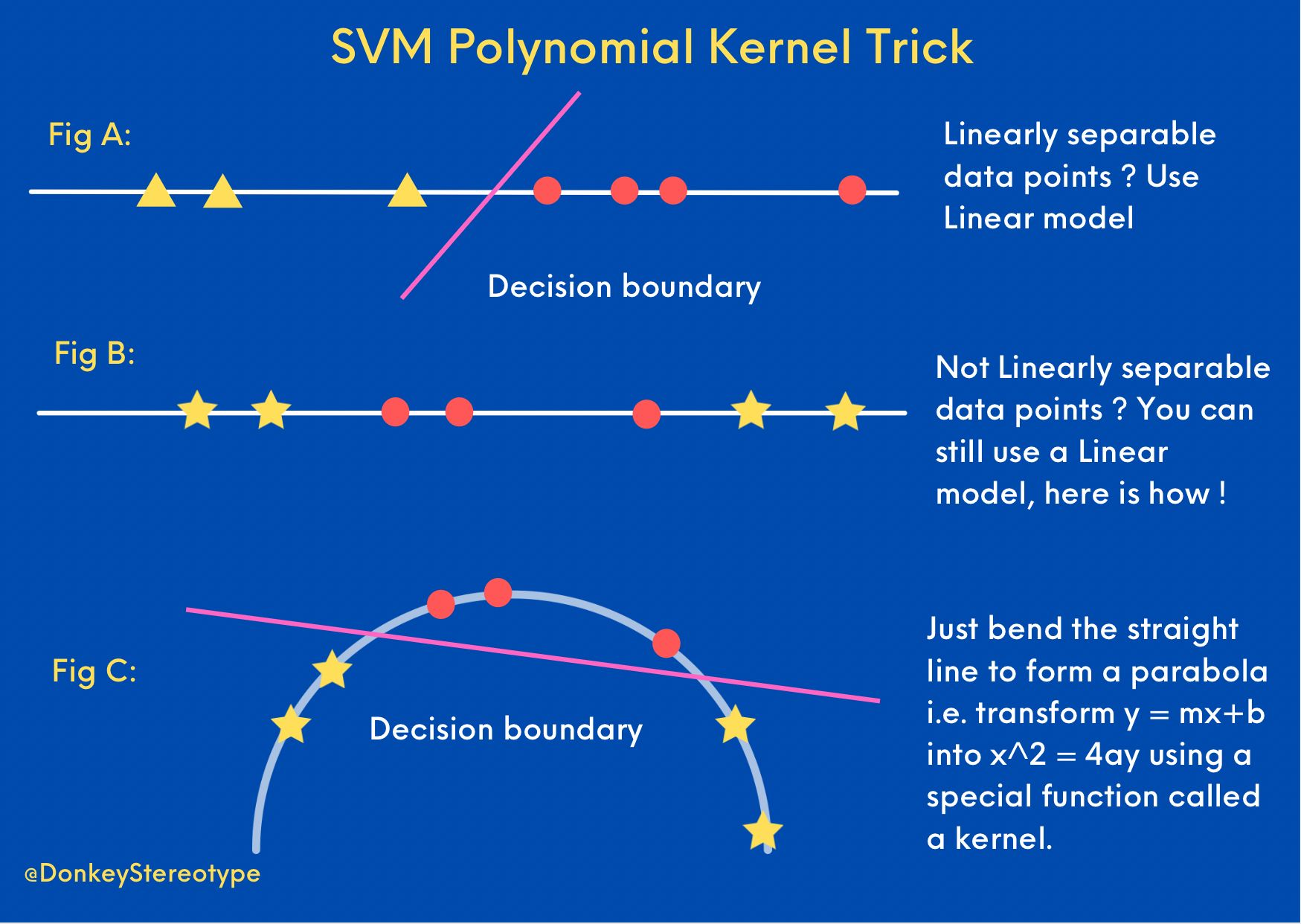## Kernel/Polynomial Trick

• In classic mathematical induction, the goal of a classification task is to learn a function $$y = f(x; \theta)$$ aka fit a model (or a curve) to the data with a decision boundary that separates the classes (say, approve/reject loan applications).

• The diagram below from Prithvi Da summarizes the approaches.• Fig A: If the data points are linearly separable, it’s simple you can fit a linear model using algorithms like Logistic regression or SVM.

• Fig B: What do you do if the data points are NOT linearly separable? of course we can fit a Neural network which in theory can learn any Borel measurable function under the sky. But we have a simple solution especially if you have a smaller tabular dataset.

• Fig C: Shows you can simply magically transform a line to a parabola and then learn a function that linearly separates the data points. This idea of applying a non-linear transformation using a special function called a “kernel” to convert linear input space ($$y= mx+c$$, 1st-degree polynomial) to a high degree polynomial ($$x^2 = 4ay$$, 2nd-degree polynomial) is the gist of the kernel trick. The bottom-line is that learning a linear model in the higher-dimensional space is equivalent to learning a non-linear model in the original lower-dimensional input space.

• TL;DR - It pays in spades to bend the problem, literally sometimes.

## Citation

If you found our work useful, please cite it as:

@article{Chadha2020DistilledKernelTrick,
title   = {Kernel Trick},